# [PYTHON] I got lost in the maze

Good evening (* ´ω ｀) Thank you for your continued support.

This time is a maze. Let's see how many moves you need from Start to Goal.

The sample maze below is Thank you for borrowing the heritage of an expert m (_ _) m

#### `maze.py`

``````
# "#"Is a wall
# "S"Is the start point,"G"Is the Goal point
# "."Is the way
maze = [
['#', 'S', '#', '#', '#', '#', '#', '#', '.', '#'],
['.', '.', '.', '.', '.', '.', '#', '.', '.', '#'],
['.', '#', '.', '#', '#', '.', '#', '#', '.', '#'],
['.', '#', '.', '.', '.', '.', '.', '.', '.', '.'],
['#', '#', '.', '#', '#', '.', '#', '#', '#', '#'],
['.', '.', '.', '.', '#', '.', '.', '.', '.', '#'],
['.', '#', '#', '#', '#', '#', '#', '#', '.', '#'],
['.', '.', '.', '.', '#', '.', '.', '.', '.', '.'],
['.', '#', '#', '#', '#', '.', '#', '#', '#', '.'],
['.', '.', '.', '.', '#', '.', '.', '.', 'G', '#'],
]
``````

The above has commas between the elements, It's hard to understand because there is space. Let's make the display a little easier to understand. Specifically, I tried to display it again with the following description.

#### `maze.py`

``````
def print_maze(maze):
for xx in maze:
for yy in xx:
print(yy,end="")
print()

print_maze(maze)

#Execution result
#S######.#
......#..#
.#.##.##.#
.#........
##.##.####
....#....#
.#######.#
....#.....
.####.###.
....#...G#

``````

After that, while saying that I will get lost in the maze, It is assumed that the coordinates of Start and Goal are known in advance.

#### `maze.py`

``````
sx, sy = 0, 1 #Coordinates of starting point
gx, gy = 9, 8 #Coordinates of goal point
``````

Since it is difficult to imagine just the maze mentioned above, After all I will rewrite the maze neatly.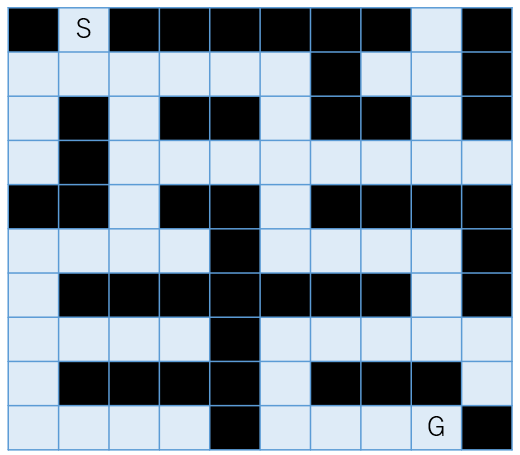Black will be the wall, so follow the light blue road from "S" to "G". Basically, we choose the coordinates that will be the "road", but there are some caveats.

** 1. I can't walk through the wall ** ** 2. The road you took once can never be taken **

1 seems to be okay if you know the composition of the maze, Regarding 2, it seems impossible unless you make a note of where you passed. So you need to have a note as well as a map of the maze, as shown in the figure below.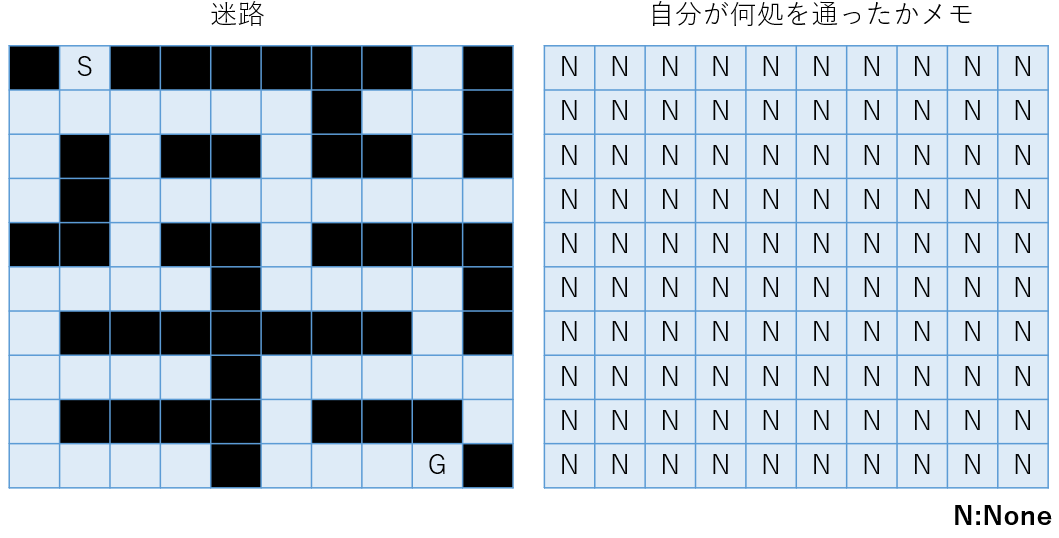I will check how many hands I got from the start to the goal, so Make a note of the count number at the coordinates you walked and proceed.

Therefore, if you return the number in the memo when you reach the goal, the mission is over. It's a selfish image, but is it like this?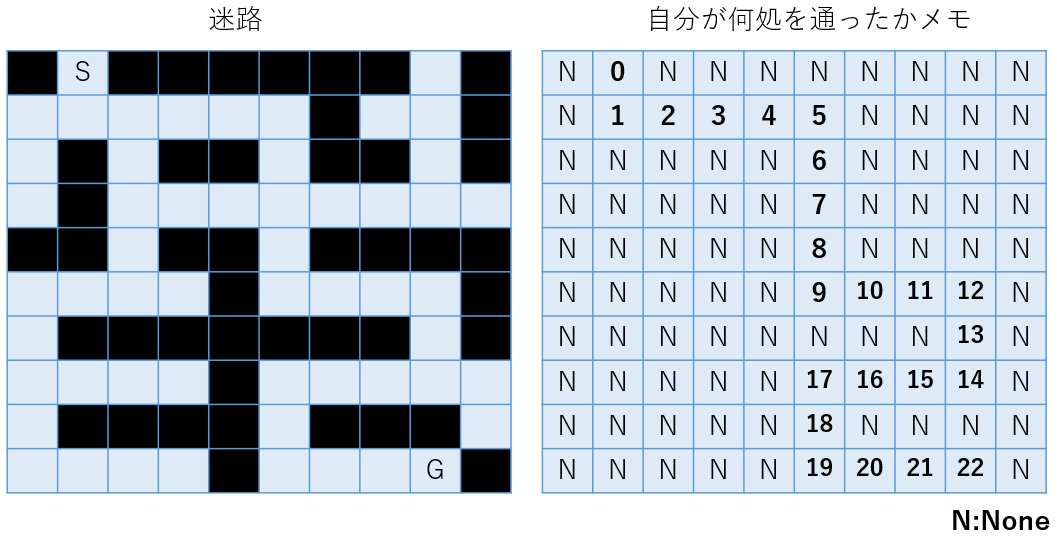Set aside how to proceed I would like to describe up to the point of making a memo.

#### `maze.py`

``````
def print_maze(maze):
for xx in maze:
for yy in xx:
print(yy,end="")
print()

def make_memo():
none = None
field_x_length = len(maze) #Number of elements in the x direction
field_y_length = len(maze)    #Number of elements in the y direction

# [ None for i in range(4)]If you try something like that, you can see the meaning of the following description
distance = [[none for i in range(field_x_length)] for j in range(field_y_length)]
print_maze(distance)

maze = [
['#', 'S', '#', '#', '#', '#', '#', '#', '.', '#'],# <== maze
['.', '.', '.', '.', '.', '.', '#', '.', '.', '#'],# <== maze
['.', '#', '.', '#', '#', '.', '#', '#', '.', '#'],# <== maze
['.', '#', '.', '.', '.', '.', '.', '.', '.', '.'],# <== maze
['#', '#', '.', '#', '#', '.', '#', '#', '#', '#'],# <== maze
['.', '.', '.', '.', '#', '.', '.', '.', '.', '#'],# <== maze
['.', '#', '#', '#', '#', '#', '#', '#', '.', '#'],# <== maze
['.', '.', '.', '.', '#', '.', '.', '.', '.', '.'],# <== maze
['.', '#', '#', '#', '#', '.', '#', '#', '#', '.'],# <== maze
['.', '.', '.', '.', '#', '.', '.', '.', 'G', '#'],# <== maze
]

print_maze(maze)
make_memo()
``````

#### `Execution result.py`

``````
#maze: print_maze(maze)
#S######.#
......#..#
.#.##.##.#
.#........
##.##.####
....#....#
.#######.#
....#.....
.####.###.
....#...G#

#Note: make_memo()
NoneNoneNoneNoneNoneNoneNoneNoneNoneNone
NoneNoneNoneNoneNoneNoneNoneNoneNoneNone
NoneNoneNoneNoneNoneNoneNoneNoneNoneNone
NoneNoneNoneNoneNoneNoneNoneNoneNoneNone
NoneNoneNoneNoneNoneNoneNoneNoneNoneNone
NoneNoneNoneNoneNoneNoneNoneNoneNoneNone
NoneNoneNoneNoneNoneNoneNoneNoneNoneNone
NoneNoneNoneNoneNoneNoneNoneNoneNoneNone
NoneNoneNoneNoneNoneNoneNoneNoneNoneNone
NoneNoneNoneNoneNoneNoneNoneNoneNoneNone
``````

Now let's move on to the fields we have prepared. !?

Yes, it's a field. When you say a field, of course, you have coordinates, right? (That? A little forcible !? (ﾟ Д ﾟ)) I tried to shake the coordinates a little.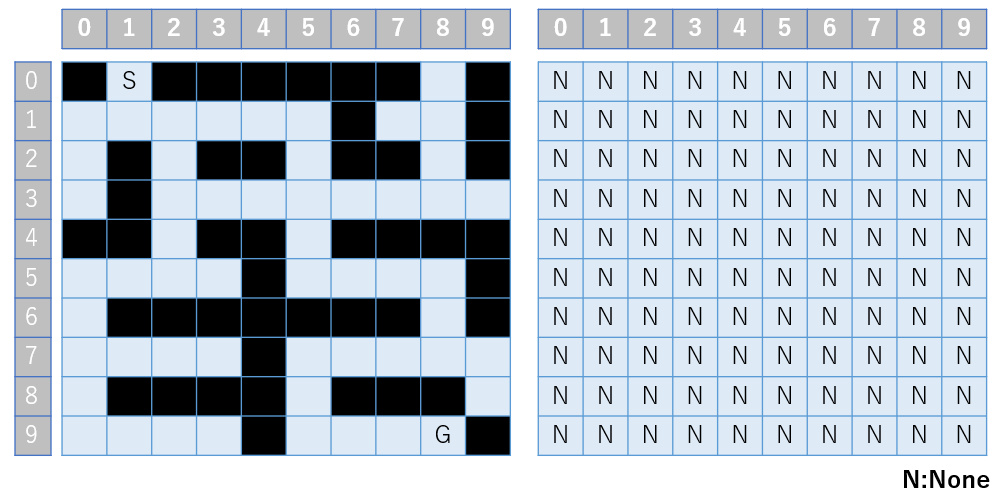You can't do anything without coordinates to make a note of where and how to proceed Well, it may be natural to define.

The rest is the direction to go. For example, if → (right), it is (x: 1, y: 0) considering the above coordinates, isn't it? Below is a summary of how to proceed in all four directions.

** → Right (x: 1, y: 0) ** ** ← Left (x: -1, y: 0) ** ** ↑ Above (x: 0, y: -1) ** ** ↓ Below (x: 0, y: 1) **

Wouldn't it be nice if the coordinates when all the above were turned in the for statement from a certain point satisfy the following conditions?

1. Not a wall
2. Do not jump out of the defined coordinates
3. A place I've never been to

Let's write it right away !!

#### `maze.py`

``````
for i in range(4):#Set to 4 to specify up, down, left, and right
#nx,ny :Coordinates of destination, x,y :Current coordinates
nx, ny = x + [1, 0, -1, 0][i], y + [0, 1, 0, -1][i] # nx,ny = x + X[i], y + Y[i]Is equivalent to
# i = 0 : nx , ny = x + 1, y + 0 ....→ Right(x: 1,y: 0)
# i = 1 : nx , ny = x + 0, y + 1 ....↑ Above(x: 0,y:-1)
# i = 2 : nx , ny = x +-1, y + 0 ....← Left(x:-1,y: 0)
# i = 3 : nx , ny = x + 0, y +-1 ....↓ Below(x: 0,y: 1)

#   |←-----Do not jump out the horizontal axis x-----→|　　|←-----Do not jump out of the vertical axis y-----→|
if (0 <= nx and nx < field_x_length and 0 <= ny and ny < field_y_length \
#|←-The wall does not break through!-→|   |←-----First place!------→|
and maze[nx][ny] != '#' and distance[nx][ny] == none):
#distance[x][y]:Current location+A value of 1 is distance[nx][ny]Note to
distance[nx][ny] = distance[x][y] + 1
``````

The rest starts from the start until you reach the goal If you repeat the above process, you will know how many moves you have reached the goal. Hate (...? How do you control it? .. ..

When in trouble, as mentioned above Move what you want to do (program) that you wrote quickly Let's plot the operation. I'm sure you'll see what's missing.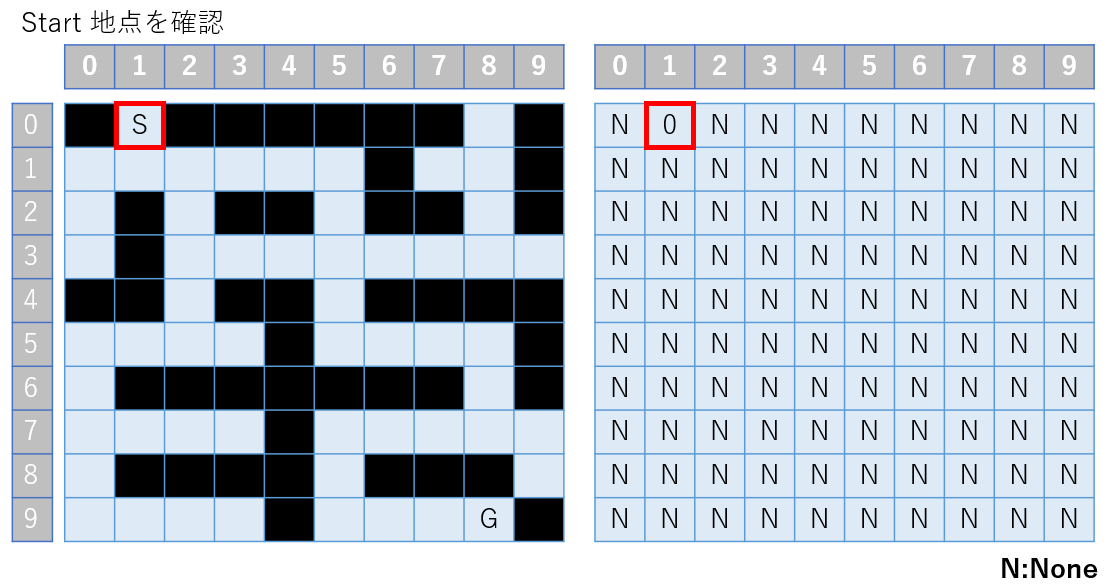There is no problem here. Let's move up, down, left and right from here. At that time, considering the above-mentioned restrictions, what should we do? .. ..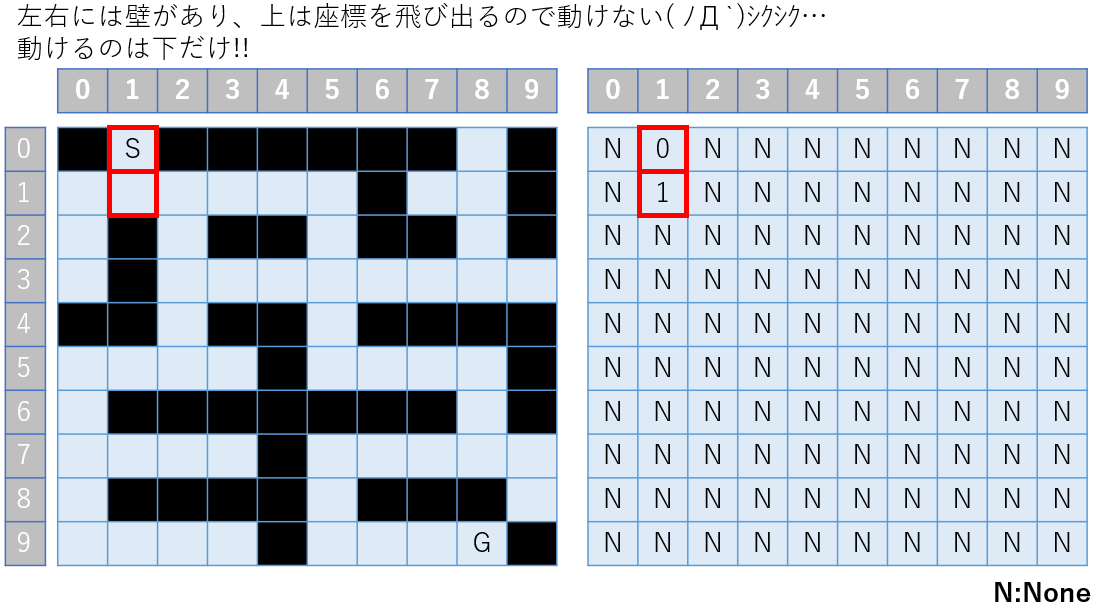Let's try up, down, left and right in the next square.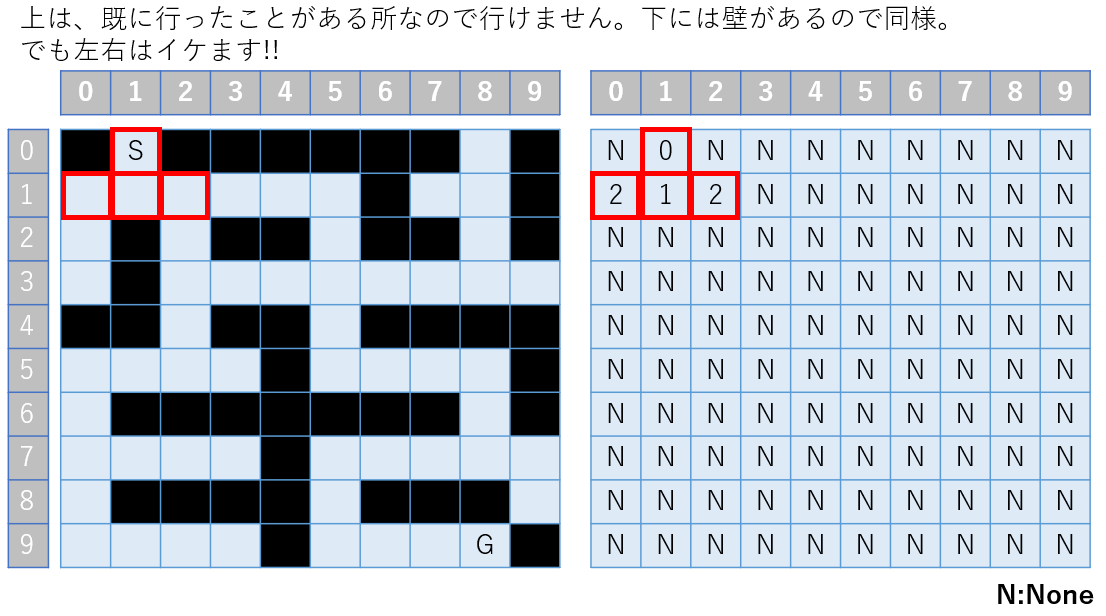Oh !? I've split into two. It became necessary to perform processing that moves in all directions at the coordinates of [0,1] and [2,1]. Is it possible to handle the branch by buffering and processing each case? This time we will use a queue. There is a reason for this.

In the previous Let's face the partial sum, if a branch point is created, I chose either path and went all the way to the dead end (depth-first search). But by fasting in and fasting out the coordinates of the branch point as a queue, You can process both coordinates of the branch point and then process the next lower layer, right? As shown below, the image is red => blue => green and each layer is processed and then processed toward the lower layer.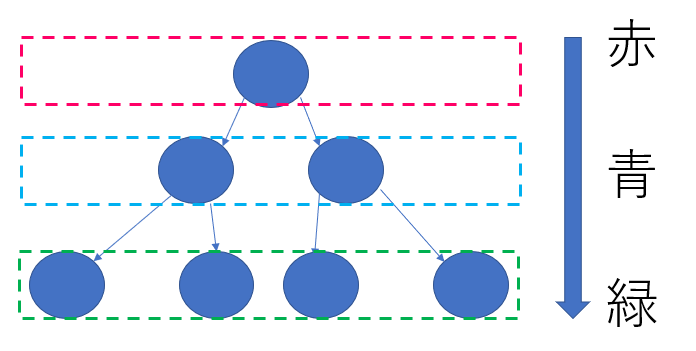It seems that this is called ** breadth-first search **. Conversely, if you change the queue to a stack, it will be a depth-first search. If it is difficult to understand or if it is wrong, please comment m (_ _) m

Now, let's say you're lucky and you're just before the goal. Is it like this at that time?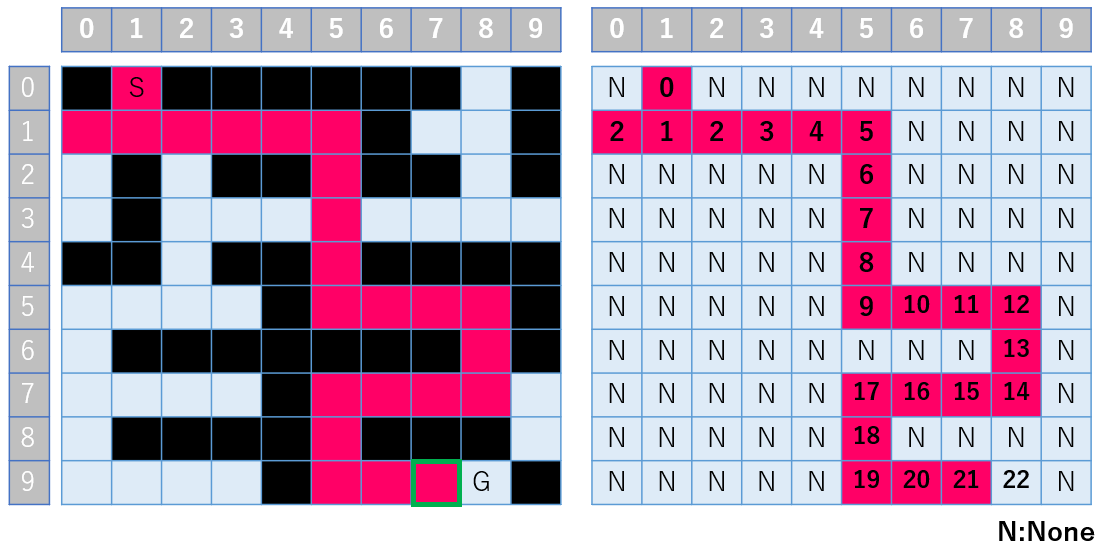** * Because it is troublesome, only the shortest route is painted in red. Actually, the branching is repeated, so the squares on the road (light blue) should turn red m (_ _) m **

Look at the green frame in the upper left figure. As long as you arrive just before the goal, it is natural that you have to go to the right, but the point is ** It means that the length of data buffered in the queue will never be 0 even just before the goal **. From the above, the process of deciding the direction of travel and proceeding is repeated with a while statement. You just have to break when you reach a certain coordinate.

#### `maze.py`

``````
def solve_maze(sx, sy, gx, gy, maze):

none = None
field_x_length = len(maze)
field_y_length = len(maze)

distance = [[none for i in range(field_x_length)] for j in range(field_y_length)]

buff = []#Buffer coordinates

distance[sx][sy]=0#Rewrite None in the start coordinate to 0

while len(buff):# len(buff) ==Loop until 0
x,y = buff.pop(0)#Update your current location
if x == gx and y == gy:#break when you reach the goal
break
for i in range(4):
nx, ny = x + [1, 0, -1, 0][i], y + [0, 1, 0, -1][i]
if (0 <= nx and nx < field_x_length and 0 <= ny and ny < field_y_length \
and maze[nx][ny] != '#' and distance[nx][ny] == none):
buff.append([nx,ny])#Buffer the coordinates that are likely to advance
distance[nx][ny] = distance[x][y] + 1
#Show the final result of the memo
print_maze(distance)
#Finally, return the memo of the goal point
return distance[gx][gy]
``````

#### `Execution result.py`

``````
#Memo result
None0NoneNoneNoneNoneNoneNone13None
212345None1312None
3None3NoneNone6NoneNone11None
4None4567891011
NoneNone5NoneNone8NoneNoneNoneNone
8767None9101112None
9NoneNoneNoneNoneNoneNoneNone13None
10111213None1716151415
11NoneNoneNoneNone18NoneNoneNone16
12131415None19202122None

#Distance to the goal
22
``````

I'm sorry, it's hard to understand, so I'll draw it.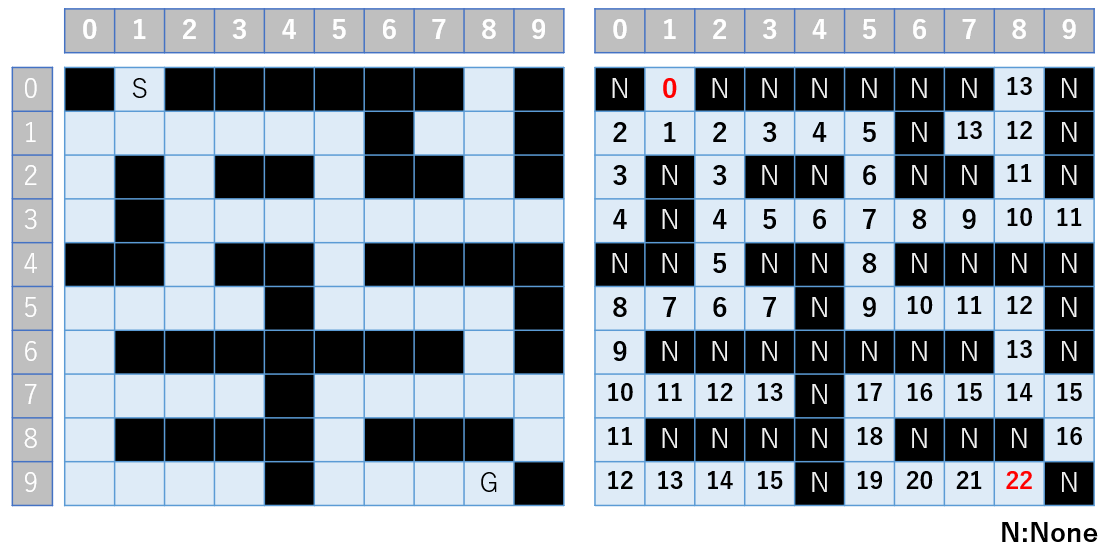Programs can do this (..) φ memo memo I learned ('◇') ゞ